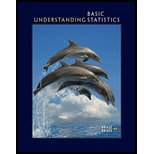# Political Science: Voters This problem is based on information taken from The Rating Guide to Life in America's Fifty States, by G. S. Thomas. A random sample of n 1 = 288 voters registered in the state of California showed that 141 voted in the last general election. A random sample of n 2 = 216 registered voters in the state of Colorado showed that 125 voted in the most recent general election. Do these data indicate that the population proportion of voter turnout in Colorado is higher than that in California? Use a 5% level of significance.### Understanding Basic Statistics

8th Edition
Charles Henry Brase + 1 other
Publisher: Cengage Learning
ISBN: 9781337558075

#### Solutions

Chapter
Section### Understanding Basic Statistics

8th Edition
Charles Henry Brase + 1 other
Publisher: Cengage Learning
ISBN: 9781337558075
Chapter 10.3, Problem 10P
Textbook Problem
3 views

## Political Science: Voters This problem is based on information taken from The Rating Guide to Life in America's Fifty States, by G. S. Thomas. A random sample of n 1 =   288 voters registered in the state of California showed that 141 voted in the last general election. A random sample of n 2 =   216 registered voters in the state of Colorado showed that 125 voted in the most recent general election. Do these data indicate that the population proportion of voter turnout in Colorado is higher than that in California? Use a 5% level of significance.

(a)

To determine

The level of significance and state the null and alternative hypotheses.

### Explanation of Solution

The level of significance, α=0.05. Let p1 be the population proportion of voter turnout in Californiaand p2 be the proportion population proportion ofvoter turnout in Colorado

(b)

To determine

The sampling distribution to be used and explain the assumptions and also find the value of the sample test statistic.

(c)

To determine

To find: The P-value of the sample statistic and sketch the sampling distribution and show the area corresponding to the P-value.

(d)

To determine

Whether we reject or fail to reject the null hypothesis and whether the data statistically significant at level α.

(e)

To determine

To explain: The conclusion in the context of application.

### Still sussing out bartleby?

Check out a sample textbook solution.

See a sample solution

#### The Solution to Your Study Problems

Bartleby provides explanations to thousands of textbook problems written by our experts, many with advanced degrees!

Get Started

Find more solutions based on key concepts
2. Define the terms population, sample, parameter and statistic.

Statistics for The Behavioral Sciences (MindTap Course List)

63-78 Evaluate the limit. limxln(100x2)

Calculus (MindTap Course List)

In Exercises 4756, solve the given equation for the indicated variable. 5x+1=1125

Finite Mathematics and Applied Calculus (MindTap Course List)

In problems 17-30, simplify each expression so that only positive exponents remain. 27.

Mathematical Applications for the Management, Life, and Social Sciences

In Exercises 16, find the points of intersection of the graphs of the functions. Express your answer accurate t...

Applied Calculus for the Managerial, Life, and Social Sciences: A Brief Approach

Evaluate the integral 34. (arcsinx)2dx

Single Variable Calculus: Early Transcendentals

Finding Intercepts In Exercises 19-28, find any intercepts. x2yx2+4y=0

Calculus: Early Transcendental Functions (MindTap Course List)

Let f(x)={2x2forx13forx1. Which limit does not exist? a) limx3f(x) b) limx1+f(x) c) limx1f(x) d) limx1f(x)

Study Guide for Stewart's Single Variable Calculus: Early Transcendentals, 8th